Tamil Nadu Board of Secondary EducationTamil Nadu Primary School Class 4th
Advertisement Remove all ads

# Samacheer Kalvi solutions for Mathematics Term 3 - Class 4th Tamil Nadu State Board chapter 3 - Measurements [Latest edition]

Advertisement Remove all ads

#### Chapters

Chapter 1: Geometry

Chapter 2: Numbers

Chapter 3: Measurements

Chapter 4: Time

Chapter 5: Money

Chapter 6: Fraction## Chapter 3: Measurements

Exercise 3.1Exercise 3.2Exercise 3.3Exercise 3.4
Exercise 3.1 [Pages 20 - 21]

### Samacheer Kalvi solutions for Mathematics Term 3 - Class 4th Tamil Nadu State Board Chapter 3 Measurements Exercise 3.1 [Pages 20 - 21]

Exercise 3.1 | Q 1 | Page 20

Rani had 1 litre coconut oil. She shared it equally among her 5 friends. How much does each person have?

Exercise 3.1 | Q 2 | Page 20

A teapot contains 2 litres, it is poured in cups with a capacity of 500 ml. How many cups can be filled?

Exercise 3.1 | Q 3 | Page 20

Ram has 1 litre of juice bottle if he gives his friend 100 ml of juice. How much is left with him?

Exercise 3.1 | Q 4.1 | Page 21

Change litre into millilitre.

1 l = ______ ml

Exercise 3.1 | Q 4.2 | Page 21

Change litre into millilitre.

7 l = ______ ml

Exercise 3.1 | Q 4.3 | Page 21

Change litre into millilitre.

5 l = ______ ml

Exercise 3.1 | Q 4.4 | Page 21

Change litre into millilitre.

9 l = ______ ml

Exercise 3.1 | Q 4.5 | Page 21

Change litre into millilitre.

4 l = _________ ml

Exercise 3.1 | Q 4.5 | Page 21

Change litre into millilitre.

4 l = ______ ml

Exercise 3.1 | Q 5.1 | Page 21

Change millilitre into litre

6000 ml = ______ l

Exercise 3.1 | Q 5.2 | Page 21

Change millilitre into litre

2000 ml = ______ l

Exercise 3.1 | Q 5.3 | Page 21

Change millilitre into litre

8000 ml = ______ l

Exercise 3.1 | Q 5.4 | Page 21

Change millilitre into litre

9000 ml = ______ l

Advertisement Remove all ads
Exercise 3.2 [Pages 22 - 23]

### Samacheer Kalvi solutions for Mathematics Term 3 - Class 4th Tamil Nadu State Board Chapter 3 Measurements Exercise 3.2 [Pages 22 - 23]

Exercise 3.2 | Q 1. i) | Page 22

Complete the following.

5 l + 376 ml = ______ ml + ______ ml = ______ ml

Exercise 3.2 | Q 1. ii) | Page 22

Complete the following.

3 l + 735 ml = ______ ml + ______ ml = ______ ml

Exercise 3.2 | Q 1. iii) | Page 22

Complete the following.

4 l + 43 ml = ______ ml + ______ ml = ______ ml

Exercise 3.2 | Q 1. iv) | Page 22

Complete the following.

8 l + 6 ml = ______ ml + ______ ml = ______ ml

Exercise 3.2 | Q 1. v) | Page 22

Complete the following.

6 l + 800 ml = ______ ml + ______ ml = ______ ml

Exercise 3.2 | Q 2 | Page 23

Match the following measurements given in the bottels with the measurements in the boxes given below the bottels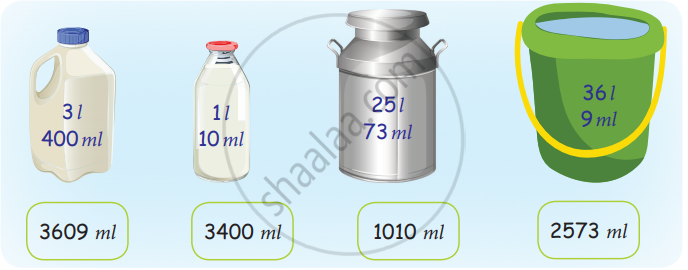Advertisement Remove all ads
Exercise 3.3 [Pages 24 - 25]

### Samacheer Kalvi solutions for Mathematics Term 3 - Class 4th Tamil Nadu State Board Chapter 3 Measurements Exercise 3.3 [Pages 24 - 25]

Exercise 3.3 | Q I. 1. | Page 24

Add the following:

l     ml
25  830
(+) 42  126

Exercise 3.3 | Q I. 2 | Page 24

Add the following:

l     ml
13  645
(+) 54  143

Exercise 3.3 | Q I. 3. | Page 24

Add the following:

l     ml
13  250
(+) 32  140

Exercise 3.3 | Q II. 1. | Page 24

Subtract the following:

l     ml
15   400
(−)  7   300

Exercise 3.3 | Q II. 2. | Page 24

Subtract the following:

l     ml
29   910
(−) 21   500

Exercise 3.3 | Q II. 3. | Page 24

Subtract the following:

l     ml
63   560
(−) 34   230

#### Solve the following

Exercise 3.3 | Q III. 1. | Page 25

Ramu filled the petrol in his two cars. The first one hold the capacity of 23 l 500 ml and the second one hold the capacity 15 l 750 ml. Find the total quantity of petrol

Exercise 3.3 | Q III. 2. | Page 25

Kannan has some cows. They give milk in the first week 48 l 480 ml and in the second week 57 l 530 ml. Find the total capacity of milk

Exercise 3.3 | Q III. 3. | Page 25

The fruit juice used in a function are given below.

 S. No Cool drinks Capacity 1. Apple juice 16 l 500 ml 2. Mango juice 23 l 160 ml 3. Grapes juice 19 l 650 ml 4. Lemon juice 20 l 350 ml

Find the total quantity of juice used in the above table.
1. Apple juice + Lemon juice = _____________ l ____________ ml
2. Mango juice + Grapes juice = ____________ l ___________ ml
3. Lemon juice + Mango juice = ____________ l ___________ ml

Exercise 3.3 | Q III. 4. | Page 25

The shop keeper had 43 l 750 ml of groundnut oil. He sold 24 l 350 ml. How much oil was left with him?

Exercise 3.3 | Q III. 5. | Page 25

A bucket contains 15 l 500 ml of water. Gopi poured out 5 l 200 ml to water the plants. How much water is left in the bucket?

Exercise 3.3 | Q III. 6. | Page 25

I purchased 73 l of milk. My sister has taken 34 l 500 ml of milk. So how much milk is left for me?

Exercise 3.3 | Q III. 7. | Page 25

Find the difference between these two cans.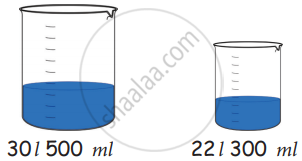Advertisement Remove all ads
Exercise 3.4 [Page 27]

### Samacheer Kalvi solutions for Mathematics Term 3 - Class 4th Tamil Nadu State Board Chapter 3 Measurements Exercise 3.4 [Page 27]

Exercise 3.4 | Q 1). i) | Page 27

Estimate how much liquid object can hold.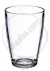Can hold (l/ml) ______ of milk.

• 500 ml

• 100 ml

• 50 ml

• 25 l

• 20 l

Exercise 3.4 | Q 1). ii) | Page 27

Estimate how much liquid object can hold.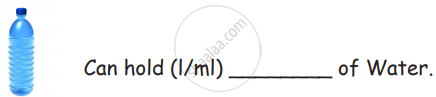• 500 ml

• 100 ml

• 50 ml

• 25 ml

• 20 ml

Exercise 3.4 | Q 1). iii) | Page 27

Estimate how much liquid object can hold.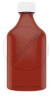Can hold (l/ml) ______ of syrup.

• 500 ml

• 100 ml

• 50 ml

• 25 ml

• 20 l

Exercise 3.4 | Q 1). iv) | Page 27

Estimate how much liquid object can hold.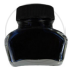Can hold (l/ml) ______ of ink.

• 500 ml

• 100 ml

• 50 ml

• 25 ml

• 20 ml

Exercise 3.4 | Q 1). v) | Page 27

Estimate how much liquid object can hold.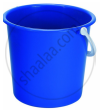can hold (l/ml) ______ of water.

• 500 ml

• 100 ml

• 50 ml

• 25 ml

• 20 l

Advertisement Remove all ads

## Chapter 3: Measurements

Exercise 3.1Exercise 3.2Exercise 3.3Exercise 3.4## Samacheer Kalvi solutions for Mathematics Term 3 - Class 4th Tamil Nadu State Board chapter 3 - Measurements

Samacheer Kalvi solutions for Mathematics Term 3 - Class 4th Tamil Nadu State Board chapter 3 (Measurements) include all questions with solution and detail explanation. This will clear students doubts about any question and improve application skills while preparing for board exams. The detailed, step-by-step solutions will help you understand the concepts better and clear your confusions, if any. Shaalaa.com has the Tamil Nadu Board of Secondary Education Mathematics Term 3 - Class 4th Tamil Nadu State Board solutions in a manner that help students grasp basic concepts better and faster.

Further, we at Shaalaa.com provide such solutions so that students can prepare for written exams. Samacheer Kalvi textbook solutions can be a core help for self-study and acts as a perfect self-help guidance for students.

Concepts covered in Mathematics Term 3 - Class 4th Tamil Nadu State Board chapter 3 Measurements are Measuring Volume of Given Liquid Using Containers Marked with Standard Units., Estimation of the Volume of a Liquid Contained in a Vessel and Verification by Measuring.

Using Samacheer Kalvi Class 4th solutions Measurements exercise by students are an easy way to prepare for the exams, as they involve solutions arranged chapter-wise also page wise. The questions involved in Samacheer Kalvi Solutions are important questions that can be asked in the final exam. Maximum students of Tamil Nadu Board of Secondary Education Class 4th prefer Samacheer Kalvi Textbook Solutions to score more in exam.

Get the free view of chapter 3 Measurements Class 4th extra questions for Mathematics Term 3 - Class 4th Tamil Nadu State Board and can use Shaalaa.com to keep it handy for your exam preparation

Advertisement Remove all ads
Share
Notifications

View all notifications

Forgot password?